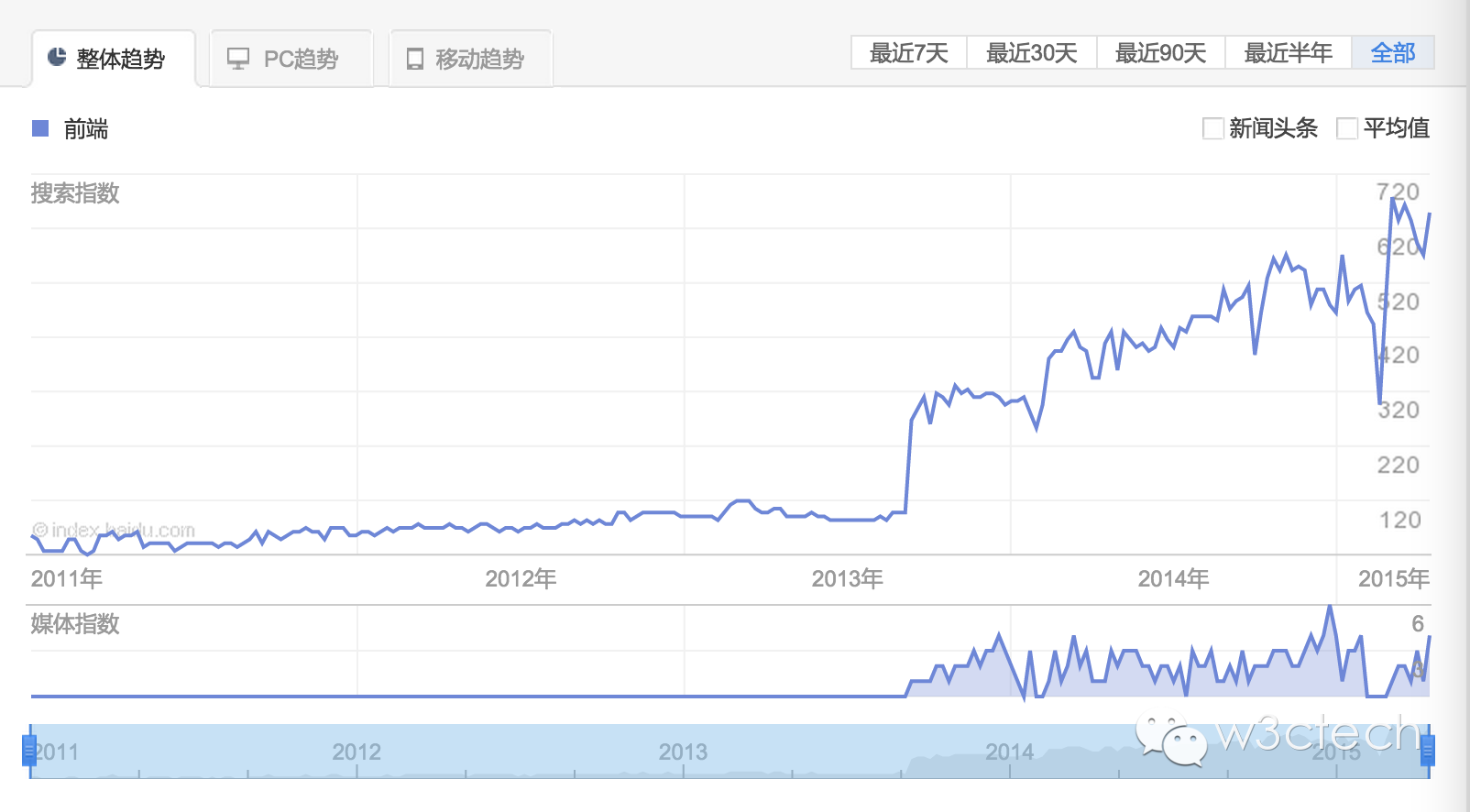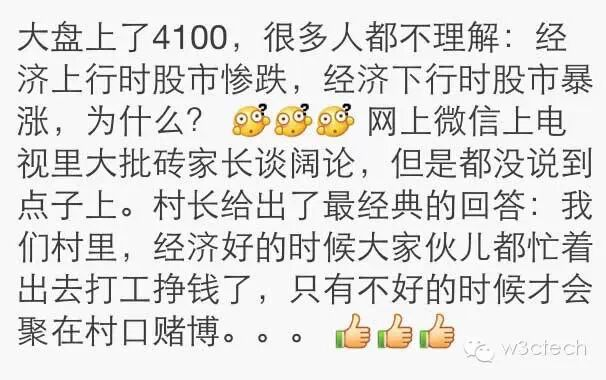# 【第17期】别慌！这里是前端！

• 十年踪迹
• 7725 访问

http://www.w3ctech.com/topic/916

http://dongfei.baijia.baidu.com/article/52449

http://www.w3ctech.com/topic/922``````function turn(color){
traffic.className = color;
}

function wait(d){
return new Promise(function(resolve, reject) {
setTimeout(resolve,d);
})
}

void function(){
Promise.resolve()
.then(turn.bind(null,'green'))
.then(wait.bind(null,5000))
.then(turn.bind(null,'yellow'))
.then(wait.bind(null,2000))
.then(turn.bind(null,'red'))
.then(wait.bind(null,5000))
.then(arguments.callee)
}();
``````

``````var Task = {
define: function(func){
return function(){
var self = this;
var args = [].slice.call(arguments);

var _next = this._next || new Promise(function(resolve, reject){
resolve();
});

this._next = _next.then(function(){
return new Promise(function(resolve, reject){
func.apply(self, args.concat(resolve, reject));
});
});
return this;
}
}
};

traffic.className = color;
\$resolve();
}),
setTimeout(\$resolve, time);
}),
func();
\$resolve();
})
}

//执行！
void function repeat() {
.turn('green')
.wait(5000)
.turn('yellow')
.wait(2000)
.turn('red')
.wait(5000)
.do(repeat);
}();
``````

``````function turn(color) {
traffic.className = color;
return new Promise(function(resolve) {
setTimeout(function() { resolve(this.next) }.bind(this), this.wait)
}.bind(this))
}

turn.and = turn.bind; // 仅为了可读性
void function (){
Promise.resolve('green')
.then(turn.and({wait: 5000, next: 'yellow'}))
.then(turn.and({wait: 2000, next: 'red'}))
.then(turn.and({wait: 5000}))
.then(arguments.callee)
}();
``````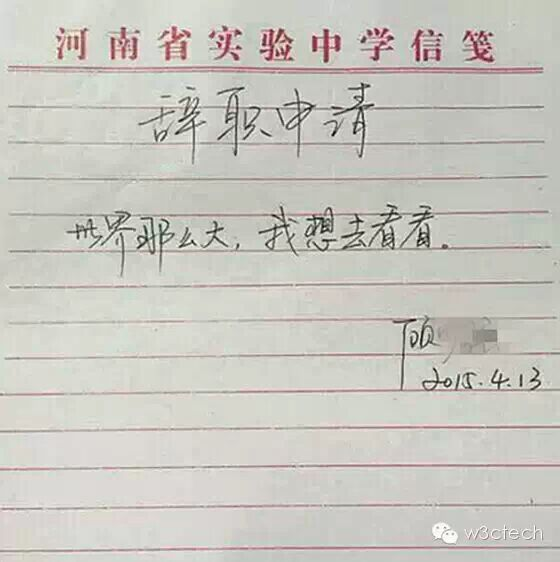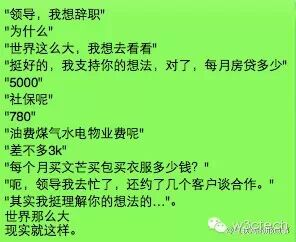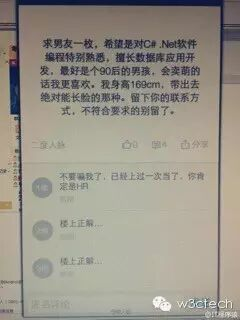``````#!/usr/local/bin/perl
# The Sieve of Eratosthenes - 埃拉托斯芬筛法
use Lingua::Sinica::PerlYuYan;

``````A股的牛市真来了，而前端的大牛市早在2013年中就来了